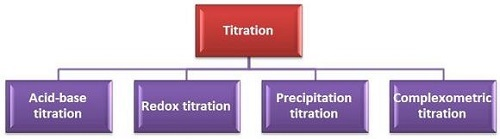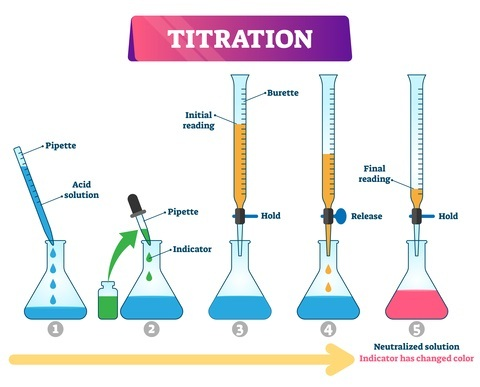# Types of Titration

## Introduction

Titration is a quantitative analysis used in chemistry used to identify the strength or concentration of a given unknown chemical solution by analysing it with a known solution concentration with the presence of an indicator.

Both oxidation and reduction happen at the same time. Titration is a process for calculating the quantity of one unknown solution concentration by other solutions. An Indicator is required to detect the endpoint of equivalence. It generally occurs with colour change.

## What is Titration?

Chemical reaction occurs with the interchange of ions when certain chemical species are in contact with each other. Titration is a quantitative analysis used in chemistry used to identify the strength or concentration of a given unknown chemical solution by analysing it with a known solution concentration with the presence of an indicator. The known concentration participating in the process of titration is called titrant. Titrated substance indicates the substance whose quantity is to be calculated.

An unknown analyte concentration needs to be added by calibrated titrant concentration” for the identification of the titration endpoint as part of the analysis. The mol count of analyte and titrant will be identical at the endpoint. Titration is helpful for volumetric analysis of different concentrations.

## Titration Types

Titration offers a volumetric analysis of solutions. It initiates the estimated volume of solution concentration in an appropriate solvent.Figure 1: types of titration

The volume estimation process or titration can be classified into four major types. These are: complexometric titration, precipitation titration, redox titration, and acid-base titration. Different titration processes are discussed below.

## Titration of Acid and Base

Acid-Base titration occurs between bases and acids, with one being the titrant and other being the titrated. The two concentrations can neutralize each other at the endpoint and produces water and salt. The equation is as follows −

$$\mathrm{A^- + H^++ B^+ + OH^-\:\rightarrow\:A^- +B^+ +H^+ + OH^–}$$

The equivalent point of acid-base titration is indicated by the pH indicator or acid-base indicator.

## Titration Procedure

• The burette, pipette and conical flask are rinsed with the standard solution, unknown solution and distilled water.

• The analyte needs to be with the accurate measurement using a pipette to send to the flask with some indicator drops.

• The burette should be filled half by the standardized solution. In addition, the measured volume needs to be recorded.

• The estimated amount of known solution is required to keep at this level.

• The solution is drained from the burette allowing the indicator colour change at a point and the value needs to be noted.

• It is the initial titration, the same procedure is done for three more times, and the average value should be noted.

• The difference of initial and final values indicates the total used titrant amount.

• The permanent colour change of indicator denotes the reach of the endpoint.Figure 2: Acid-base titration procedure

The indicators are weak basic or weak acid in nature. The indicator drops are added to the solution one by one for this titration process and neutralization is indicated with a sharp colour change. Methyl orange and Phenolphthalein are two mostly used acid-base indicators.

## Redox Titration

This titration is also known as a reaction of reduction and oxidation. Aqueous solution of reacting ions receives electrons in hemical reactions of this titration process. This titration is classified into the following types based on the applied reagents.

### Dichromate Titrations

In this reaction, the use of potassium dichromate occurs in acidic medium as an oxidising agent. Dilute sulphuric acid is used for maintaining the acidic nature of the medium. The reaction is −

$$\mathrm{K_2Cr_2O_7+4H_2SO_4\:\rightarrow\:K_2SO_4+Cr_2(SO_4)_3+4H_2O+3[O]}$$

Potassium dichromate solution is directly used to estimate iodides and ferrous salts.

### Permanganate Titrations

In this titration, the oxidizing agent is potassium permanganate. Dilute sulphuric acid is used for its maintenance. The equation is −

$$\mathrm{2KMnO_4+3H_2SO_4\:\rightarrow\:K_2SO_4+2MnSO_4+3H_2O+5[O]}$$

The solution has no colour before it reaches the endpoint. Estimation of ferrous salts, acidic acids, oxalates, and hydrogen peroxide can be done with standardized forms of potassium permanganate.

### Iodometric and Iodimetric Titrations

In this process, free iodine is reduced to iodide ions, and iodide ions are oxidised afterreleasing electrons. The expressions are −

$$\mathrm{l_2+2e^{−}\:\rightarrow\:2l^{−} }$$

$$\mathrm{2l^{−}\:\rightarrow\:I_2+2e^{−}}$$

In iodometric titration, the use of free iodines as indicators as well as an oxidation agent for the reaction and in iodometric titration to generate free iodine.

## Complexometric and Precipitation Titrations

Precipitation titration occurs with the contact of two reacting substances with the formation of insoluble precipitate. In sodium chloride or ammonium thiocyanate solutions, the use of silver nitrate solution proceeds with the formation of white precipitate or silver chloride or silver thiocyanate after reactions. The reaction is expressed as −

$$\mathrm{AgNO_3+NaCl\:\rightarrow\:AgCl+NaNO_3}$$

In complexometric titrations, the formation of an undissociated complex occurs at the point of equivalence. Co-precipitations in this process do not give errors. It is more useful than precipitation titrations method. The expression complexometric titration process is asfollows −

$$\mathrm{Hg^{2+}+2SCN^{−}\:\rightarrow\:Hg(SCN)_2}$$

Some metal complexes are formed with the help of a useful reagent is Ethylenediaminetetraacetic acid.

## Conclusion

Titration is the quantity measurement process of one substance with the presence of another substance. The substance whose quantity is calculated called titrated and the titrant exists with the known concentrations.

Titration can be divided into different types based on the reaction participant types. These are, acid-base titrations, precipitation titrations, redox titrations, and complexometric titrations. Phenolphthalein, methyl-orange and other indicators are used for identifying the endpoint of the titration process.

## FAQs

Q1. What are indicators in titration?

Ans. Indicators are weak acid and weak base substances that make their colour change while identifying chemical changes in solutions. They indicate the change of pH level in a solution that they are participating in.

Q2. Why titration is important?

Ans. Titration helps to understand the concentration quantity of a solution with other acidic solutions and the solutions can be reutilized for accurately determining the concentration level.

Q3. What is the meaning of titration endpoint?

Ans. Titration endpoint is the moment when the required reactant is added in full to a solution in the titration process. It is the terminal point of titration.

Updated on: 17-Apr-2023

1K+ Views